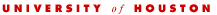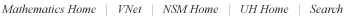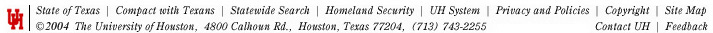ColloquiumFor further information, or to suggest a colloquium speaker, please contact the organizer.To subscribe to the Colloquium mailing-lists, please email the organizer.Roland Roeder

IUPUI

The Ising Model for magnets and the mysterious Lee-Yang zeros

Oct. 19, 2016
3:00 pm    PHG 646

 Abstract The Ising Model was developed by Lenz and Ising in the 1920s to describe magnetic materials. It is the most fundamental model of such materials, but there are still many open questions about it that continue to challenge present day physicists and mathematicians. In suitable variables, the Ising model can be described by a single polynomial $$Z(z,t)$$ of two variables $$z$$ and $$t$$ that is called the "'partition function". Lee and Yang proved in 1952 that if $$t \in [0,1]$$, then the zeros of the partition function lie on the unit circle $$|z| = 1$$. Most of the physical properties of the magnet are determined by the location of these "Lee-Yang zeros". In this talk, I will explain the Ising model, the Lee-Yang Theorem, and describe several interesting results and open questions about the locations of the Lee-Yang zeros. I will conclude by describing my joint work with Pavel Bleher and Mikhail Lyubich on the Lee-Yang zeros for the Diamond Hierarchical Lattice.Webmaster   University of Houston    ---    Last modified:  April 11 2016 - 18:14:43